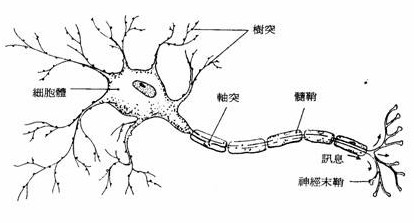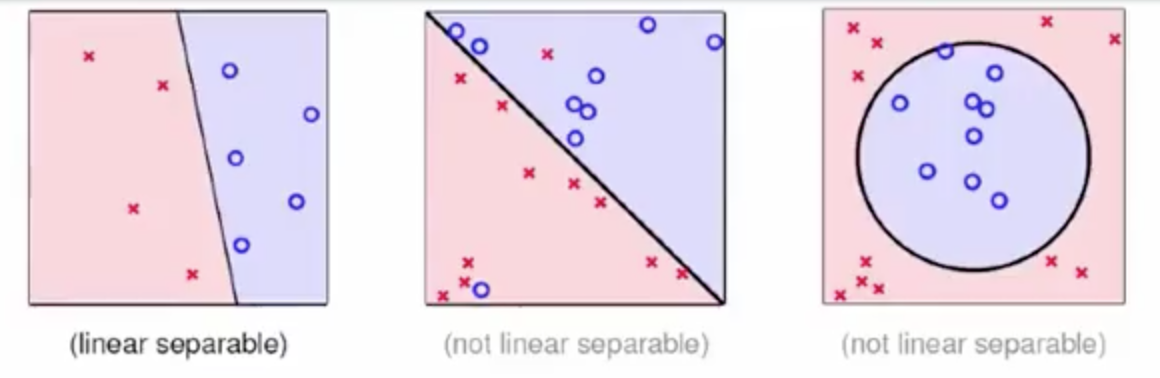## 感知器 Perceptron## Perceptron Hypothesis Set

$X = \{x_1, x,2, ... ,x_d\}$

$W = \{w_1,w_2,...,w_d\}$

$score = \sum_{i=1}^{d}{w_ix_i} , and \left\{ \begin{array}{l} score > T ,\ approve \\ score < T , \ reject \\ score = 0 ,\ ignored \end{array} \right .$

$h(x) = sign(\sum_{i=1}^{d}{w_ix_i} - T) ,\ where\ h(x) ∈ H$

$sign(x) = \left\{ \begin{array}{l} +1,\ x >0 \\ -1,\ x < 0 \end{array} \right .$

\begin{aligned} h(x) &= sign(\sum_{i=1}^{d}{w_ix_i} - T) \\ &= sign(\sum_{i=1}^{d}{w_ix_i} + \underbrace{(-T)}_{w_0} \times \underbrace{(+1)}_{x_0}) \\ &= sign(\sum_{i=0}^{d}{w_ix_i}) \\ &= sign(W^TX) \end{aligned}

### Perceptrons in $R^2$Perceptrons in R^2（圖片來源: 課程截圖

## Perceptron Learning Algorithm (PLA)

### 如何找到最適合的線段?

$W$ 其實是直線方程的法向量

### PLA 演算法

1. 初始化權重 $W$ 為 $W_0$，設定 $W_0$ = 0
2. 按序或隨機遍歷所有資料，也就是二維平面上所有的點，找出分類結果與真實標籤不符的資料：
1. 若存在，則更新權重 $W$ 後，重新執行步驟二。
2. 若不存在，停止執行。

$W_{t+1} \leftarrow W_t + Y_{n(t)}X_{n(t)}$1. 這演算法何時會停下來？
2. 如果停下來，找到的 $g_f$ 真的接近 $f$ 嗎？

## Guarantee of PLA

PLA 演算法停止必須滿足訓練集所有樣本都是線性可分的（linear separable），也就是說平面上必須至少存在一條線的，並且使的線的一側全為藍點，另一側全為紅點。### [證明] PLA 會停止運行

##### TODO: 證明改成英文的， LaTeX 搭中文好醜
1. 評估 $W_{t+1}$ 及 $W_{t}$ 與 $W_f$ 的相近程度

$\because$ $D$ 為線性可分
$\therefore$ 必存在一條直線其法向量為 $W_f$ ，使 $D$ 中所有點皆符合 $Y_n = sign(W_f^TX_n)$

$\because$ 已知存在一條法向量為 $W_f$ 的直線且 $Y_n = sign(W_f^TX_n)$
$\because$ 可知平面上任一點皆與法向量為 $W_f$ 的直線存在一定距離
$\therefore$ 故推知 $Y_nW_f^TX_n > 0$ 且 $min( Y_nW_f^TX_n) > 0$

$\because$ 在運行 $t$ 次時，存在一個分類錯誤的點 $(X_{n(t)}, Y_{n(t)})$
$\because$ 又 $(X_{n(t)}, Y_{n(t)}) \in D$
$\therefore$ 可推知 $Y_{n(t)}W_f^TX_{n(t)} \geq\ min( Y_nW_f^TX_n) > 0$

為評估 $W_f$ 與 $W_{t+1}$ 的相近程度，故使向量內積

\begin{aligned} W_f^TW_{t+1} &= W_f^T( W_t + Y_{n(t)}X_{n(t)} ) \qquad \because\ W_{t+1} \leftarrow W_t + Y_{n(t)}X_{n(t)} \\ &= W_f^TW_t + W_f^TY_{n(t)}X_{n(t)} ) \\ &\geq W_f^TW_t + min( Y_nW_f^TX_n) \qquad \because\ Y_{n(t)}W_f^TX_{n(t)} \geq\ min( Y_nW_f^TX_n) \\ &> W_f^TW_t + 0 \qquad \because\ min( Y_nW_f^TX_n) > 0 \end{aligned}

可得 $W_f^TW_{t+1} > W_f^TW_t$ ，向量內積隨執行次數增加而增加。

1. 向量內積的增長可能原因有二：向量長度增加或向量夾縮小。為排除向量長度增長對向量內積增加的影響，進行下列證明：

$\because$ 在運行 $t$ 次時，存在一個分類錯誤的點 $(X_{n(t)}, Y_{n(t)})$ ，使的 $sign(W_t^T, X_{n(t)}) \neq Y_{n(t)}$

$\therefore$ 可推知 $Y_{n(t)}(W_t^T, X_{n(t)}) \leq 0$

$\because$ 已知 $W_{t+1} \leftarrow W_t + Y_{n(t)}X_{n(t)}$ ，故可得

\begin{aligned} { \parallel W_{t+1} \parallel}^2 &= {\parallel W_t + Y_{n(t)}X_{n(t)} \parallel} ^2 \\ &= {\parallel W_t \parallel} ^2 + 2 W_tY_{n(t)}X_{n(t)} + {\parallel Y_{n(t)}X_{n(t)} \parallel} ^2 \\ &\leq {\parallel W_t \parallel} ^2 + 0 + {\parallel Y_{n(t)}X_{n(t)} \parallel} ^2 \qquad \because Y_{n(t)}(W_t^T, X_{n(t)}) \leq 0 \\ &\leq {\parallel W_t \parallel} ^2 + {max\parallel Y_{n(t)}X_{n(t)} \parallel} ^2 \\ &\leq {\parallel W_t \parallel} ^2 + {max\parallel X_{n(t)} \parallel} ^2 \qquad \because Y_{n(t)} 為 \pm1，取平方後無影響 \\ \end{aligned} \\

可得 ${ \parallel W_{t+1} \parallel}^2 \leq {\parallel W_t \parallel} ^2 + {max\parallel X_{n(t)} \parallel } ^2$ ，證明在運行中向量長度增加緩慢

1. 證明隨執行次數增加而 $W_{t+1}$ 與 $W_f$ 逐漸靠近
$\because$ 已知向量內積公式為 $\vec a \cdot \vec b = |\vec a||\vec b| cos{\theta}$
$\because$ 又從(1)中得知，向量內積隨執行次數增加而增加
$\because$ 又從(2)中得知，在運行中向量長度增加緩慢
$\therefore$ 向量內積的增加，為 $cos{\theta}$ 增加所導致
$\therefore$ 證明隨執行次數增加而 $W_{t+1}$ 與 $W_f$ 逐漸靠近

1. 證明PLA會停止
設定初始向量為 $W_0 = 0$，從 0 開始進行 $T$ 次迭代

由(2)推知：

\begin{aligned} { \parallel W_T \parallel}^2 &\leq {\parallel W_{T-1} \parallel} ^2 + {max\parallel X_{n} \parallel} ^2 \\ &\leq {\parallel W_{T-2} \parallel} ^2 + {max\parallel X_{n} \parallel} ^2 +{ max \parallel X_{n} \parallel} ^2 \\ &\leq {\parallel W_{0} \parallel} ^2 + T \cdot {max\parallel X_{n} \parallel} ^2 \\ &\leq T \cdot {max\parallel X_{n} \parallel} ^2 \qquad \because\ W_{0} = 0 \\ \end{aligned}

由(1)推知：

\begin{aligned} W_f^TW_{T} &\geq W_f^TW_{T-1}+ min( Y_nW_f^TX_n) \\ &\geq W_f^TW_{T-2}+ min( Y_nW_f^TX_n) + min( Y_nW_f^TX_n) \\ &\geq W_f^TW_{0}+ T \cdot min( Y_nW_f^TX_n) \\ &\geq T \cdot min( Y_nW_f^TX_n) \\ \end{aligned}

結合上述兩結論，可得

\begin{aligned} \frac{W_f^T}{ \parallel W_f\parallel} \frac{W_T}{ \parallel W_T \parallel} &\geq \frac {T \cdot min( Y_nW_f^TX_n)}{\parallel W_f\parallel \parallel W_T \parallel} \\ &\geq \frac {T \cdot min( Y_nW_f^TX_n)}{\parallel W_f\parallel \sqrt{T \cdot {max\parallel X_{n(t)} \parallel } ^2 }} \\ &\geq \frac {\sqrt{T} \cdot min( Y_nW_f^TX_n)}{\parallel W_f\parallel max\parallel X_{n(t)} \parallel } \\ &\geq \sqrt{T} \frac { min( Y_nW_f^TX_n)}{\parallel W_f\parallel max\parallel X_{n(t)} \parallel } \\ &\geq \sqrt{T} \cdot C , \qquad C = \frac { min( Y_nW_f^TX_n)}{\parallel W_f\parallel max\parallel X_{n(t)} \parallel } \\ \end{aligned} \\

已知內積中正規化後，乘積最多為 1
故可得

\begin{aligned} 1 &\geq \frac{W_f^T}{ \parallel W_f\parallel} \frac{W_T}{ \parallel W_T \parallel} \\ & \geq \sqrt{T} \frac { min( Y_nW_f^TX_n)}{\parallel W_f\parallel max\parallel X_{n(t)} \parallel } \\ \end{aligned}

化簡後

$\frac{R^2}{\rho^2} = \frac {\parallel W_f\parallel^2 max\parallel X_{n(t)} \parallel^2 } { min( Y_nW_f^TX_n)^2} \geq T$

其中

$R^2 = max\parallel X_{n(t)} \parallel^2 \qquad \qquad \rho^2 = min(Y_n \frac {W_f^T}{\parallel W_f\parallel }X_n)$

從最後一條式子看來T是有上限的，因此在線性可分的情況下，PLA 最終會停止

## Non-Separable Data

PLA 演算法的優缺點相當清楚，優點是簡易實做，可以適用於任何維度，缺點是資料必須是線性可分的，可是是否為線性可分通常無法事前得知。即使資料是線性可分的，但因為時間複雜度高，執行時間也會耗費相當久。

$W_g = argmin \sum_{n=1}^N (Y_n \neq sign(W^TX_n)$

1. 初始化權重 $W$ 為 $W_0$，設定 $W_0$ = 0
2. 隨機遍歷所有資料，找出分類結果與真實標籤不符的資料：
1. 若存在，則更新 $W$ 後，並統計新線段存在的錯誤點數。若總數小於所記錄，則更新記錄與對應權重。
2. 若不存在，停止執行。
3. 執行前，請先設定中止條件，如:執行次數、錯誤點少於多少時停止…等。

## 課堂測驗

Q1 . Assume that each email is represented by the frequency of keyword occurrence, and output +1 indicates a spam. Which keywords below shall have large positive weights in a

1. coffee, tea, hamburger, steak
2. free, drug, fantastic, deal
3. machine, learning, statistics, textbook
4. national, Taiwan, university, coursera

Q2 . Let $n = n(t)$, according to the rule of PLA below, which formula is true?

$sign(w^T_tx_n)≠y_n ,\ and\ ,\ , w_{t+1} \leftarrow w_t + y_nx_n$
1. $w^T_{t+1}x_n = y_n$
2. $sign(w^T_{t+1}x_n)=y_n$
3. $y_nw^T_{t+1}x_n \geq y_nw^T_tx_n$
4. $y_nw^T_{t+1}x_n < y_nw^T_tx_n$

Q3 . Define $R^2 = max_n \parallel x_n\parallel^2$ , $\rho = min_ny_n \frac {w^T_f}{\parallel x_f \parallel} x_n$. We want to show that $T \leq □$. Express the upper bound $□$ by the two terms above.

1. $R/\rho$
2. $R^2/\rho^2$
3. $R/\rho^2$
4. $\rho^2/R^2$

Q4 .Since we do not know whether $D$ is linear separable in advance, we may decide to just go with pocket instead of PLA. If $D$ is actually linear separable, what’s the difference between the two?

1. pocket on $D$ is slower than PLA
2. pocket on $D$ is faster than PLA
3. pocket on $D$ returns a better $g$ in approximating ff than PLA
4. pocket on $D$ returns a worse $g$ in approximating ff than PLA